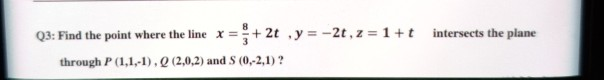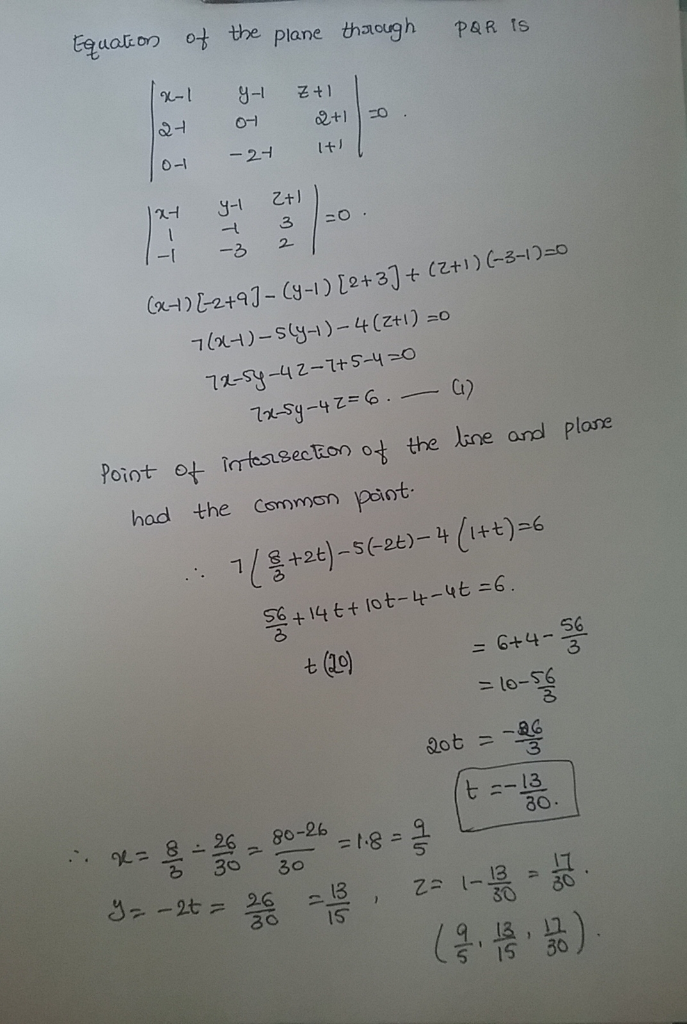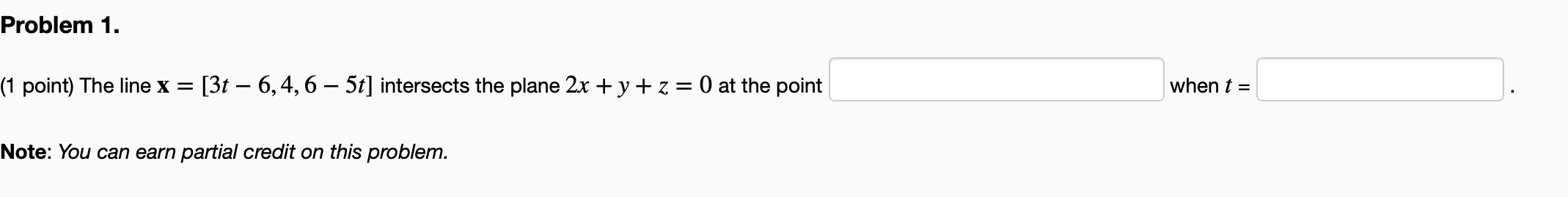# intersects the plane Q3: Find the point where the line x x = + 2t ,...intersects the plane Q3: Find the point where the line x x = + 2t , y = -2t, z = 1+t through P (1,1,-1). Q(2,0.2) and S (0,2,1) ?##### Add Answer of: intersects the plane Q3: Find the point where the line x x = + 2t ,...
Similar Homework Help Questions
• ### Find the point P

Find the point P where the line x = 1 + t, y = 2t, z = -3t intersects the plane x + y - z = 3.

• ### Help with finding a point at which the line intersects a given plane

Find the point at which the line intersects the givenplane:#44.x=1+2t, y=4t, Z=2-3t;x+2y-z+1=0

• ### a) Let L be the line through (2,-1,1) and (3,2,2). Parameterize L. Find the point Q...

a) Let L be the line through (2,-1,1) and (3,2,2). Parameterize L. Find the point Q where L intersects the xy-plane. b) Find the angle that the line through (0,-1,1) and (√3,1,4) makes with a normal vector to the xy-plane. c) Find the distance from the point (3,1,-2) to the plane x-2y+z=4. d) Find a Cartesian equation for the plane containing (1,1,2), (2,1,1) and (1,2,1)

• ### Find the equation of the plane through the point (-2,8,10) and parallel to the line x=1+t,...

Find the equation of the plane through the point (-2,8,10) and parallel to the line x=1+t, y=2t, z=4-3t

• ### Equation for the plane that passes through a pt *x,y,z*

Find an equation for the plane that passes through the point (1,-1,1) and contains the line x=3-2t, y=-t, and z=2+3t.

• ### Problem 1. (1 point) The line x = [3t – 6,4,6 – 5t] intersects the plane...Problem 1. (1 point) The line x = [3t – 6,4,6 – 5t] intersects the plane 2x + y + z = 0 at the point when t = Note: You can earn partial credit on this problem.

• ### Find an equation of the plane that contains the point P and the line. P(5,0,2); x = 3t+1, y =...

Find an equation of the plane that contains the point P and the line.P(5,0,2); x = 3t+1, y = -2t + 4, z = t-3

• ### Calculus 3 problem,

Find the point P where the line x=1+t, y=2t, z=-3t intersects the plane x+y+z=2

• ### Given   find where the line tangent to r at t=2 intersects the plane through the three points (1,0,2), (-1,0,4), and (2,...

Given   find where the line tangent to r at t=2 intersects the plane through the three points (1,0,2), (-1,0,4), and (2,-1,1) F(t)(2t2-ti tk F(t)(2t2-ti tk

• ### point where tangent plane is perpendicular

find the point on the surface x^3-2y^2+z^2=27 where the tangent plane is perpendicular to the line given parametrically as x=3t-5, y=2t+7, z=1-(sqrt2)t

Free Homework App